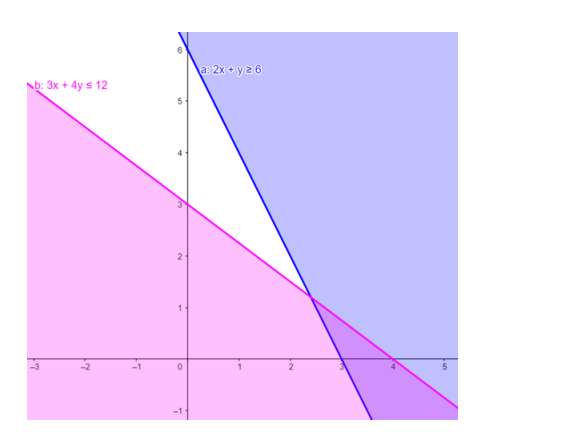# Solve the given inequalitiesQuestion:

Solve the given inequalities $2 x+y \geq 6,3 x+4 y \leq 12$ graphically in two - dimensional plane.

Solution:

The graphical representation of $2 x+y \geq 6,3 x+4 y \leq 12$ is given by common region in the figure below.

$2 x+y \geq 6 \ldots \ldots$ (1)

$3 x+4 y \leq 12 \ldots \ldots$ (2)

Inequality (1) represents the region above line $2 x+y=6$ (including the line $2 x+y=6$ ).

Inequality (2) represents the region below line $3 x+4 y=12$ (including the line $3 x+4 y=12$ ).

Therefore,every point in the common shaded region including the points on the respective lines represents the solution for the given inequalities.

This can be represented as follows,